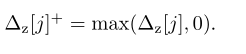# ∆z[j]+= max(∆z[j],0)

Hello, I would like to ask how to represent this in CVX, only take the number greater than 0, and the others are equal to 0;Have you looked at `pos` and `max`?

If that doesn’t suffice to enable you to express this in CVX, can you write that out in explicit (regular) MATLAB code (pretending everything were double precision MATLAB variables). I don’t understand what that notation means.